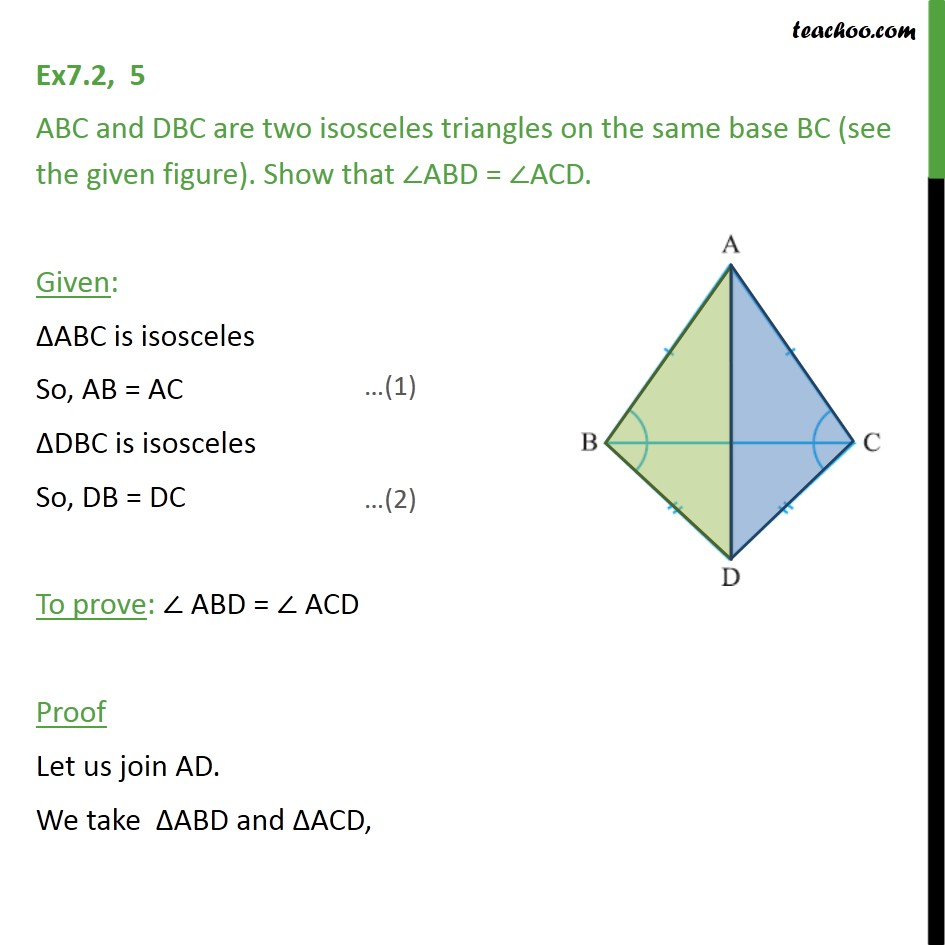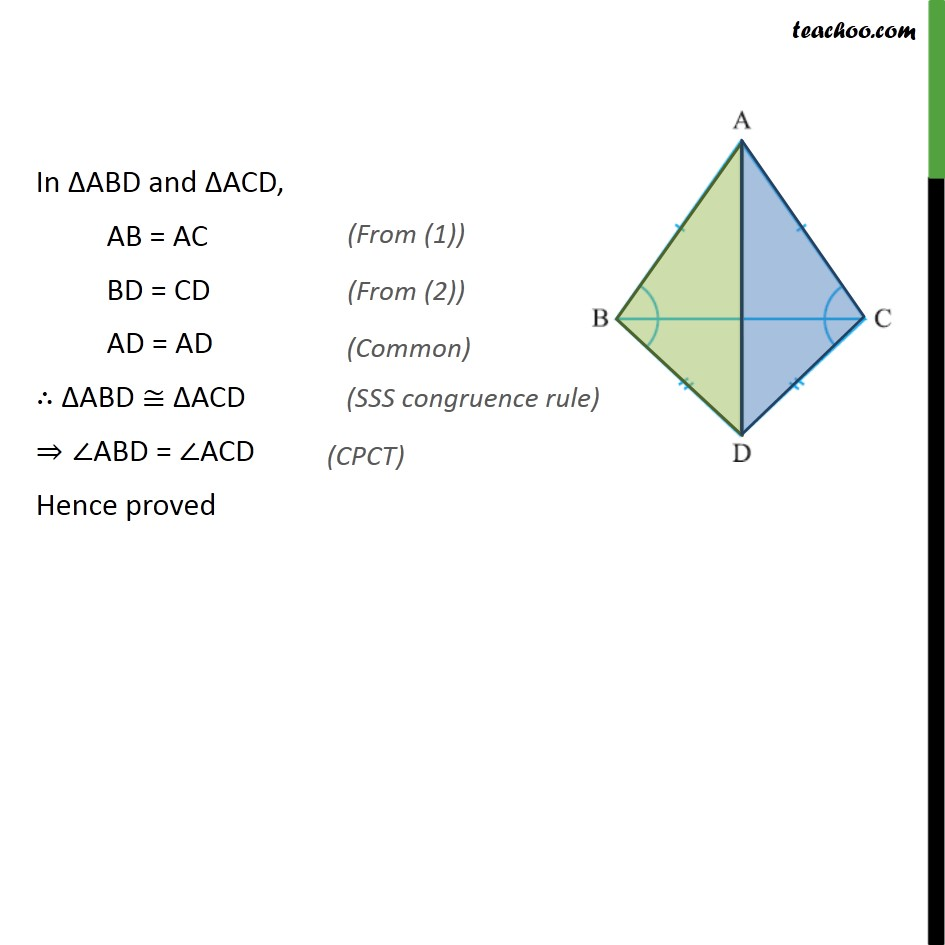Ex 7.2

Chapter 7 Class 9 Triangles
Serial order wiseLearn in your speed, with individual attention - Teachoo Maths 1-on-1 Class

### Transcript

Ex7.2, 5 ABC and DBC are two isosceles triangles on the same base BC (see the given figure). Show that ∠ABD = ∠ACD. Given: ΔABC is isosceles So, AB = AC ΔDBC is isosceles So, DB = DC To prove: ∠ ABD = ∠ ACD Proof Let us join AD. We take ΔABD and ΔACD, In ΔABD and ΔACD, AB = AC BD = CD AD = AD ∴ ΔABD ≅ ΔACD ⇒∠ABD = ∠ACD Hence proved